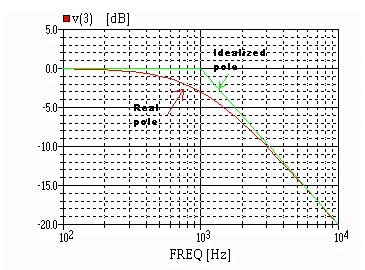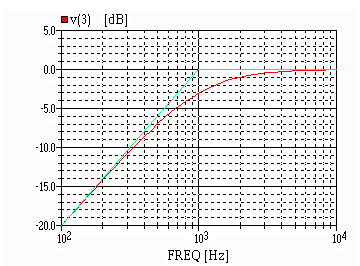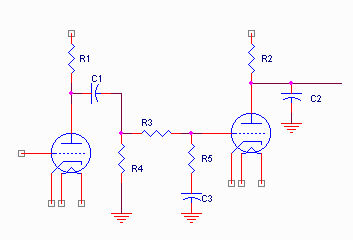Frequency Response Synthesis

Purpose

The purpose of this tutorial is to provide a method of analyzing or building circuits that alter the frequency response of an amplifier . One reason to do build such a circuit would be to provide "RIAA" phono compensation in a preamp you're considering. Another reason to do this would be to achieve "loudness" compensation. Yet another might be to compensate for specific characteristics of your speaker system. The purpose of analyzing these circuits is to gain insight into the frequency response of an existing or proposed amplifier. This is often useful before attempting to alter the response, as it provides a way of predicting what effect a proposed change might have.

This tutorial will work through some background material describing what alters frequency response, and how to apply this knowledge to several circuit examples.

Background Material

BASIC CONCEPTS -- POLES AND ZEROS: These are the "frequency domain" response manipulating concepts. A pole causes the frequency response to tip "clockwise" at 6 dB per octave (20 dB per decade). [Note: an octave is a two to one change in frequency and a decade is a ten to one change in frequency]. A zero causes the frequency response to tip "counterclockwise" at 6 dB per octave. At the "corner" frequency, the response is altered by 3 dB and by 45 degrees. (A clockwise bend is 45 degrees lag, a counterclockwise bend is 45 degrees lead). Poles can be "bought" for your intended circuit fairly cheaply, while zeros at anything other than DC usually "cost". Practically, what this means is it's easy to "roll off" response by incorporating a capacitor, but difficult to achieve a "boosted response": the boost usually means that everything EXCEPT the boost region is "cut" and the cut is selectively removed in the "boosted" region. This will be clarified later.

An example of a pole is an RC network: series resistor, capacitor to ground. This has the pole at the frequency f=1/(2*pi*r*c). It also has a zero at "infinite frequency". Its frequency response looks like this:Note the difference between the idealized pole response (green), and the frequency response actually achieved (red). The real response is 3dB down at the calculated pole's frequency. There are additional observations to be made. At exactly the "corner", the actual response differs from the idealized response by 3dB. At exactly one octave away (at 500Hz and at 2kHz in the example), the error from "ideal" is exactly 1dB. At 2 octaves away (at 250Hz and 4kHz in the example), the error from "ideal" is 0.3 dB. At 3 octaves away (at 125Hz and 8kHz in the example), the error is 0.1dB and so on. We will use this later to estimate the "synthesis" of a complex response.

A coupling network (series c, r to ground) has a zero at DC (zero frequency) and a pole at the expected f=1/(2*pi*r*c). Its frequency response looks like this:Note that in this picture, as above, the idealized pole is shown in green and the actual response is shown in red. There is also a zero at DC (causing the response to tilt up at 6dB/octave or 20dB/decade) and the pole causes the response to tilt "clockwise" causing the response to flatten.

BASIC CONCEPTS -- POLE OR ZERO ALTERATION OF FREQUENCY RESPONSE

Each pole or zero changes the slope of the frequency response curve by exactly 6 dB per octave (which is 20 dB per decade).

If the response was flat, a pole will cause the response to fall at 6dB per octave above the pole frequency. If the response was rising at 6dB per octave, a pole will cause the response to flatten above the pole frequency. If the response was falling at 6dB per octave, a pole will cause the response to fall at 12dB per octave above the pole frequency.

If the response was flat, a zero will cause the response to rise at 6 dB per octave above the zero frequency. If the response was rising at 6dB per octave, a zero will cause the response to rise at 12 dB per octave above the zero frequency. If the response was falling at 6dB per octave, a zero will cause the response to flatten above the zero frequency.

BASIC CONCEPTS -- "COST" OF POLES AND ZEROS AT "real" FREQUENCIES

1. A pole is "free". That is, it is always possible to get the response to tilt downward (or flatten if it was rising) with only passive components.

2. A zero is ALWAYS paired with a pole. That is, it is essentially impossible to get a free zero. In order to get response to tilt up, you must lower the entire level by the required "tilt up". Then you can buy a zero to tilt the response up, and the "automatic" pole will flatten the response when you have "used up" the originally dropped level. I know this is confusing: let's assume we wish to tilt up the response starting at 1 kHz and wish to have 14 dB of boost. In this case, we must first accept 14 dB of overall loss below the zero. When we get to "0dB loss" (at 5kHz in this example) there will be a pole to flatten the response again).

3. Poles CAN occur at zero frequency. This DOES require infinite gain at DC. One example is the phase comparator of a phase locked loop. A pole at DC is called a true integrator.

4. A zero may also be called a differentiator. However, since you can't buy a zero without buying a pole, there are no true differentiators. Another way of looking at this is a true differentiato would require infinite gain at infinite frequency, which also equates to infinite energy!

5. Integrators and differentiators work EXACTLY the same as their mathematical counterparts. Those of you who have taken calculus can appreciate this.

BASIC CONCEPTS -- CIRCUIT ELEMENTS FOR ALTERING FREQUENCY RESPONSE:

1. Series R, C to ground (low pass): Single pole at 1/(2*pi*R*C)

2. Series C, R to ground (high pass): Zero at DC, pole at 1/(2*pi*R*C)

3. Series R, L to ground (high pass): Zero at DC, pole at R/(2*pi*L)

4. Series L, R to ground (low pass): single pole at R/(2*pi*L)

5. Series LRC. 2 Complex poles. Presents low impedance (R) at resonance, which is at 1/(2*pi*sqrt(L*C)). Impedance increases away from resonance.

6. Parallel RLC. 2 Complex Poles. Presents high impedance (R) at resonance which is at 1/(2*pi*sqrt(L*C)). Impedance decreases away from resonance.

7. Transformer. Somewhat complicated, see: http://members.aol.com/sbench101/TubeMisc/fb_faq3.txt

We will defer discussion of complex poles and zeros to a future article.

Real world RC/RL Networks

The complication in the real world is usually associated with non-zero source impedance, and non-infinite load impedance. Consider an R-C coupling mechanism from plate of one stage to grid of the next stage. What is the frequency response? Initially, one might be tempted to just use the grid resistor as the "R" in the equation, and the coupling capacitor as the "C" in the equation. But what about the plate resistance? [The effective output resistance is the parallel combination of the plate load resistor and the plate resistance of the tube]. This value must be added to (as if it were in series with) the grid resistor value and the sum used as the "R" in the equation to determine the pole location. Yet the impedance that the grid sees (to determine the high frequency rolloff pole is the parallel combination of the output resistance and the grid resistor.

For inductively coupled circuits, you must also consider the winding resistance of the inductor. It helps in analysis to scribble on the schematic a resistor in series with the inductor or transformer whose value is the winding resistance. This resistor is NOT ADDED TO YOUR CIRCUIT, it merely comes along "free" with the inductor or transformer.

This is probably as good a point as anywhere in this tutorial to note a common mistake associated with the cathode bypass capacitor. This is really a pole-zero pair. The zero occurs at a lower frequency than the pole. Considering an increasing frequency starting from some arbitrarily low value, the "gain" of the circuit is initially the same as an unbypassed cathode circuit. As the frequency is increased the gain increases (the capacitor starts to have an effect). This is the "zero" and its frequency is associated with the cathode resistor and the bypass capacitor. As the frequency is further increased, the gain again flattens out to its midband value. This is the "pole" and the frequency associated with it is associated with the capacitor value and the PARALLEL combination of the cathode resistor and 1/gm (which is effectively the tubes cathode impedance). [to a first approximation... there are other factors, but this is the simplest "estimation" approach].

Real world R-C circuit interact with each other. For a circuit with only one reactive element (such as a single pole or a pole-zero pair), this is not too much of a problem. However, with multiple reactive elements, setting or finding the pole or zero "frequency" is not necessarily easy. In the section below, we will use an RIAA network as an example. Yet even here there is a problem. This is illustrated in the next section.

Example 1 - RIAA Network

Here's the response associated with RIAA playback equalization:As you can see, an RIAA network requires a "loss" at 1kHz of about 20 dB. The idealized pole-zero points are: a pole at 50Hz, a zero at 500Hz and another pole at 2123Hz (75uS). The first pole-zero pair is sometimes called the "turnover", and the second pole the "rolloff". Let's first consider the turnover. With a pole at 50 Hz, we would expect the real response at 50Hz to be down 3dB. However the zero at 500 Hz also affects it. (At 250 Hz, the zero  removes 1dB from the rolloff, at 125 Hz, 0.3dB, at 62.5Hz 0.1dB and at 50Hz about 0.07dB. Thus, instead of 3dB down, the response would be 2.93dB down. At 100 Hz, instead of the response being the idealized 6 dB down, the response will be 7dB down - the influence of the zero (about 0.2dB), so the response ought to be about 6.8dB down. Etc.Notice the "real response" is indeed about 6.8 dB down at 100Hz. At 500Hz, we would expect the response to be -16.93 dB (instead of -20 ideal, accounting for the 500Hz zero and the 50Hz pole), except we also have to account for the influence of the 2+kHz pole lowering the response another 0.3dB to about -17.3dB. Notice the "real" response is indeed 17.3 dB down.

Now, lets consider a circuit that produces that response.Initially, we will consider C1 to be a very large coupling capacitor. The turnover pole zero is primarily determined by R3 R5 and C3. The rolloff is determined by the second tubes plate resistance in parallel with R2, and C2.

For the turnover, we must make the voltage divider for low frequencies to middle frequencies to be 20dB (1/10).. So, I'll arbitrarily pick some values. A division of 10:1 can be achieved with 333k and 37k. (37k/(333k+37k). I'll arbitrarily choose R1 to be 100k, and R4 to be 470k. If I consider a 6SL7, the plate resistance will be about 46k, so the effective resistance in series with R3 is 46k paralleled with 100k paralleled with 470k, or about 33k. Thus, the "real" value for R3 is 300k. R5 can be 37.4k (standard 1% value). Then, the capacitive reactance of C3 must be 37k at 500Hz producing a pole at 50Hz and a zero at 500Hz. C=1/(2*pi*500*37k)=8.6nF (parallel of 4.7nF and 3.9nF)..

The rolloff for RIAA is positioned at R2. Since the plate resistance is about 45k, and assuming a plate load resistor of 100k, then this parallel combination (about 33k) requires a capacitor of 2.27nF. Considering stray capacitive loading, a 2.2nF would work well.

Modifications:

Modeling the circuit above produced a "predicted" frequency response within a tenth dB or so of the red RIAA curve above, when I used a 100 uF coupling capacitor at C1. However, decreasing this value to 0.1uF would normally be expected to alter the response only by a tenth dB or so at 20Hz. However, the response was low at 20Hz by about 1.1dB! There is that much interaction! This can be easily "compensated for" though. By increasing the value of R3 from 300k to 332k (standard 1% value), the response again followed RIAA within about 0.1dB, although the overall circuit gain drops by about a dB.

Instead of placing the rolloff capacitor (C2) on the output, is it possible to include it in the same nest as R3, R4, R5 and C3? Yes, but again some interaction occurs. C2 could be placed across R5, but to achieve accurate RIAA compensation, R5 will need to be altered. It turns out that with R5=27.4k and C2 placed across R5 and equal to 2.95nF response is again within a tenth dB of true RIAA.

To give some feel as to the "sensitivity" of the components, with the last "mod" (C2 across R5), if C2 is changed from 2.95nF to 3.05 nF, the frequencies above 3kHz will be too low by about 0.2dB, which isn't too bad.

Steve